# 最短路 & 差分约束 总结

一、引例

1、一类不等式组的解

1、Dijkstra

2、图的存储

3、链式前向星

4、Dijkstra + 优先队列

5、Bellman-Ford

6、SPFA

7、Floyd-Warshall

1、数形结合

2、三角不等式

3、解的存在性

4、最大值 => 最小值

5、不等式标准化

1、线性约束

2、区间约束

3、未知条件约束

1.Dijkstra    a.邻接矩阵实现，b.优先队列优化实现

2.Bellman-Ford

3.SPFA(FIFO队列优化)

4.Folyd

1、一类不等式组的解

给定n个变量和m个不等式，每个不等式形如 x[i] - x[j] <= a[k] (0 <= i, j < n, 0 <= k < m， a[k]已知)，求 x[n-1] - x 的最大值。例如当n = 4，m = 5，不等式组如图一-1-1所示的情况，求x3 - x0的最大值。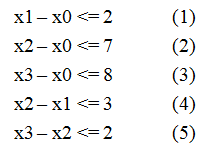观察x3 - x0的性质，我们如果可以通过不等式的两两加和得到c个形如 x3 - x0 <= Ti 的不等式，那么 min{ Ti | 0 <= i < c } 就是我们要求的x3 - x0的最大值。于是开始人肉，费尽千辛万苦，终于整理出以下三个不等式：

1.      (3)                       x3 - x0 <= 8

2.      (2) + (5)              x3 - x0 <= 9

3.      (1) + (4) + (5)     x3 - x0 <= 7

这里的T等于{8， 9， 7}，所以min{ T } = 7，答案就是7。的确是7吗？我们再仔细看看，发现的确没有其它情况了。那么问题就是这种方法即使做出来了还是带有问号的，不能确定正确与否，如何系统地解决这类问题呢？

让我们来看另一个问题，这个问题描述相对简单，给定四个小岛以及小岛之间的有向距离，问从第0个岛到第3个岛的最短距离。如图一-1-2所示，箭头指向的线段代表两个小岛之间的有向边，蓝色数字代表距离权值。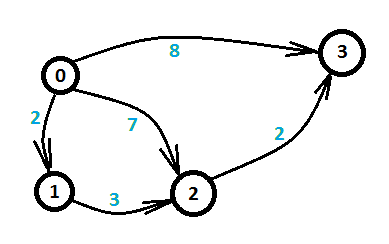这个问题就是经典的最短路问题。由于这个图比较简单，我们可以枚举所有的路线，发现总共三条路线，如下：

1.       0 -> 3                       长度为8

2.       0 -> 2 -> 3               长度为7+2 = 9

3.       0 -> 1 -> 2 -> 3       长度为2 + 3 + 2 = 7

最短路为三条线路中的长度的最小值即7，所以最短路的长度就是7。这和上面的不等式有什么关系呢？还是先来看看最短路求解的原理，看懂原理自然就能想到两者的联系了。

1、Dijkstra

对于一个有向图或无向图，所有边权为正（边用邻接矩阵的形式给出），给定a和b，求a到b的最短路，保证a一定能够到达b。这条最短路是否一定存在呢？答案是肯定的。相反，最长路就不一定了，由于边权为正，如果遇到有环的时候，可以一直在这个环上走，因为要找最长的，这样就使得路径越变越长，永无止境，所以对于正权图，在可达的情况下最短路一定存在，最长路则不一定存在。这里先讨论正权图的最短路问题。

最短路满足最优子结构性质，所以是一个动态规划问题。最短路的最优子结构可以描述为：

D(s, t) = {Vs ... Vi ... Vj ... Vt}表示s到t的最短路，其中i和j是这条路径上的两个中间结点，那么D(i, j)必定是i到j的最短路，这个性质是显然的，可以用反证法证明。

基于上面的最优子结构性质，如果存在这样一条最短路D(s, t) = {Vs ... Vi Vt}，其中i和t是最短路上相邻的点，那么D(s, i) = {Vs ... Vi} 必定是s到i的最短路。Dijkstra算法就是基于这样一个性质，通过最短路径长度递增，逐渐生成最短路。

Dijkstra算法是最经典的最短路算法，用于计算正权图的单源最短路（Single Source Shortest Path，源点给定，通过该算法可以求出起点到所有点的最短路），它是基于这样一个事实：如果源点到x点的最短路已经求出，并且保存在d[x] ( 可以将它理解为D(s, x) )上，那么可以利用x去更新 x能够直接到达的点 的最短路。即：

d[y] = min{ d[y], d[x] + w(x, y) }           y为x能够直接到达的点，w(x, y) 则表示x->y这条有向边的边权

具体算法描述如下：对于图G = <V, E>，源点为s，d[i]表示s到i的最短路，visit[i]表示d[i]是否已经确定(布尔值)。

1) 初始化 所有顶点 d[i] = INF, visit[i] = false，令d[s] = 0；

2) 从所有visit[i]为false的顶点中找到一个d[i]值最小的，令x = i; 如果找不到，算法结束；

3) 标记visit[x] = true, 更新和x直接相邻的所有顶点y的最短路： d[y] = min{ d[y], d[x] + w(x, y) }

（第三步中如果y和x并不是直接相邻，则令w(x, y) = INF）

2、图的存储

以上算法的时间复杂度为O(n^2)，n为结点个数，即每次找一个d[i]值最小的，总共n次，每次找到后对其它所有顶点进行更新，更新n次。由于算法复杂度是和点有关，并且平方级别的，所以还是需要考虑一下点数较多而边数较少的情况，接下来以图一-2-1为例讨论一下边的存储方式。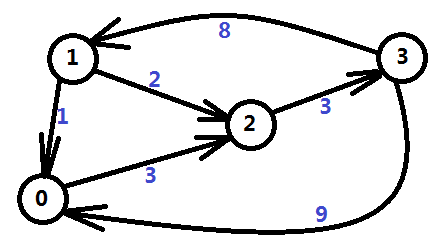邻接矩阵是直接利用一个二维数组对边的关系进行存储，矩阵的第i行第j列的值 表示 i -> j 这条边的权值；特殊的，如果不存在这条边，用一个特殊标记来表示；如果i == j，则权值为0。它的优点是实现非常简单，而且很容易理解；缺点也很明显，如果这个图是一个非常稀疏的图，图中边很少，但是点很多，就会造成非常大的内存浪费，点数过大的时候根本就无法存储。图一-2-2展示了图一-2-1的邻接矩阵表示法。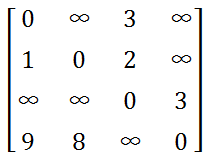邻接表是图中常用的存储结构之一，每个顶点都有一个链表，这个链表的数据表示和当前顶点直接相邻的顶点（如果边有权值，还需要保存边权信息）。邻接表的优点是对于稀疏图不会有数据浪费，缺点就是实现相对麻烦，需要自己实现链表，动态分配内存。图一-2-3展示了图一-2-1的邻接表表示法。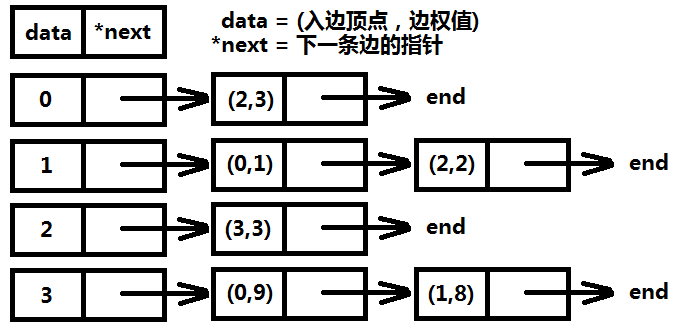前向星是以存储边的方式来存储图，先将边读入并存储在连续的数组中，然后按照边的起点进行排序，这样数组中起点相等的边就能够在数组中进行连续访问了。它的优点是实现简单，容易理解，缺点是需要在所有边都读入完毕的情况下对所有边进行一次排序，带来了时间开销，实用性也较差，只适合离线算法。图一-2-4展示了图一-2-1的前向星表示法。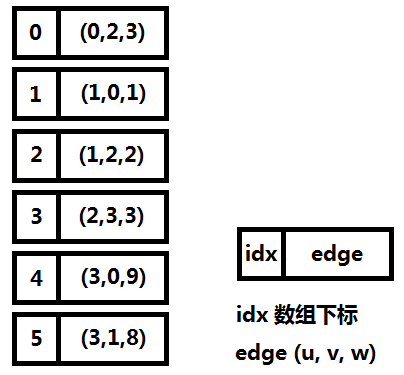那么用哪种数据结构才能满足所有图的需求呢？这里介绍一种新的数据结构一一链式前向星。

3、链式前向星

链式前向星和邻接表类似，也是链式结构和线性结构的结合，每个结点i都有一个链表，链表的所有数据是从i出发的所有边的集合（对比邻接表存的是顶点集合），边的表示为一个四元组(u, v, w, next)，其中(u, v)代表该条边的有向顶点对，w代表边上的权值，next指向下一条边。

边的结构体声明如下：

struct EDGE {
int u, v, w, next;
EDGE() {}
EDGE(int _u, int _v, int _w, int _next) {
u = _u, v = _v, w = _w, next = _next;
}
}edge[MAXM];

初始化所有的head[i] = INF，当前边总数 edgeCount = 0

void addEdge(int u, int v, int w) {
edge[ edgeCount ] = EDGE(u, v, w, head[u]);
}

这个函数的含义是每加入一条边(u, v)，就在原有的链表结构的首部插入这条边，使得每次插入的时间复杂度为O(1)，所以链表的边的顺序和读入顺序正好是逆序的。这种结构在无论是稠密的还是稀疏的图上都有非常好的表现，空间上没有浪费，时间上也是最小开销。

4、Dijkstra + 优先队列(小顶堆)

有了链式前向星，再来看Dijkstra算法，我们关注算法的第3)步，对和x直接相邻的点进行更新的时候，不再需要遍历所有的点，而是只更新和x直接相邻的点，这样总的更新次数就和顶点数n无关了，总更新次数就是总边数m，算法的复杂度变成了O(n^2 + m)，之前的复杂度是O(n^2)，但是有两个n^2的操作，而这里是一个，原因在于找d值最小的顶点的时候还是一个O(n)的轮询，总共n次查找。那么查找d值最小有什么好办法呢？

数据结构中有一种树，它能够在O( log(n) )的时间内插入和删除数据，并且在O(1)的时间内得到当前数据的最小值，这个和我们的需求不谋而合，它就是最小二叉堆(小顶堆)，具体实现不讲了，比较简单，可以自行百度。

在C++中，可以利用STL的优先队列( priority_queue )来实现获取最小值的操作，这里直接给出利用优先队列优化的Dijkstra算法的类C++伪代码（请勿直接复制粘贴到C++编译器中编译执行），然后再进行讨论：

void Dijkstra_Heap(s) {
for(i = 0; i < n; i++) {
d[i] = (i == s) ? 0 : INF;  // 注释1
}
q.push( (d[s], s) );            // 注释2
while( !q.empty() ) {
(dist, u) = q.top();        // 注释3
q.pop();                    // 注释4
for (e = head[u]; e != INF; e = edge[e].next) {
v = edge[e].v;
w = edge[e].w;
if(d[u] + w < d[v]) {
d[v] = d[u] + w;
path[v] = u;
q.push( (d[v], v) );
}
}
}
}

注释1：初始化s到i的初始最短距离，d[s] = 0

注释2：q即优先队列，这里略去声明是为了将代码简化，让读者能够关注算法本身而不是关注具体实现，   push是执行优先队列的插入操作，插入的数据为一个二元组(d[u], u)

注释3：执行优先队列的获取操作，获取的二元组为当前队列中d值最小的

注释4：执行优先队列的删除操作，删除队列顶部的元素（即注释3中d值最小的那个二元组）

以上伪代码中的主体部分竟然没有任何注释，这是因为我要用黑色的字来描述它的重要性，而注释只是注释一些和语法相关的内容。

主体代码只有一个循环，这个循环就是遍历了u这个结点的边链表，其中e为边编号，edge[e].w即上文提到的w(u, v)，即u ->v 这条边的权值，而d[u] + w(u, v) < d[v]表示从起点s到u，再经过(u, v)这条边到达v的最短路比之前其它方式到达v的最短路还短，如图二-4-1所示，如果满足这个条件，那么就更新这条最短路，并且利用path数组来记录最短路中每个结点的前驱结点，path[v] = u，表示到达v的最短路的前驱结点为u。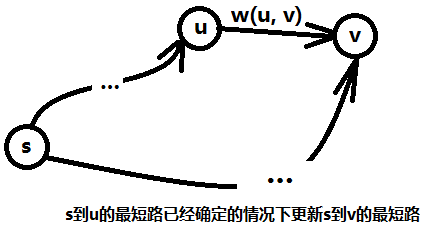补充一点，这个算法求出的是一棵最短路径树，其中s为根结点，结点之间的关系是通过path数组来建立的，path[v] = u，表明u为v的父结点（树的存储不一定要存儿子结点，也可以用存父结点的方式表示）。

考虑这个算法的复杂度，如果用n表示点数，m表示边数，那么优先队列中最多可能存在的点数有多少？因为我们在把顶点插入队列的时候并没有判断队列中有没有这个点，而且也不能进行这样的判断，因为新插入的点一定会取代之前的点（距离更短才会执行插入），所以同一时间队列中的点有可能重复，插入操作的上限是m次，所以最多有m个点，那么一次插入和删除的操作的平摊复杂度就是O(logm)，但是每次取距离最小的点，对于有多个相同点的情况，如果那个点已经出过一次队列了，下次同一个点出队列的时候它对应的距离一定比之前的大，不需要用它去更新其它点，因为一定不可能更新成功，所以真正执行更新操作的点的个数其实只有n个，所以总体下来的平均复杂度为O( (m+n)log m)，而这个只是理论上界，一般问题中都是很快就能找到最短路的，所以实际复杂度会比这个小很多，相比O(n^2)的算法已经优化了很多了。

Dijkstra算法求的是正权图的单源最短路问题，对于权值有负数的情况就不能用Dijkstra求解了，因为如果图中存在负环，Dijkstra带优先队列优化的算法就会进入一个死循环，因为可以从起点走到负环处一直将权值变小 。对于带负权的图的最短路问题就需要用到Bellman-Ford算法了。

5、Bellman-Ford

Bellman-Ford算法可以在最短路存在的情况下求出最短路，并且在存在负权圈的情况下告诉你最短路不存在，前提是起点能够到达这个负权圈，因为即使图中有负权圈，但是起点到不了负权圈，最短路还是有可能存在的。它是基于这样一个事实：一个图的最短路如果存在，那么最短路中必定不存在圈，所以最短路的顶点数除了起点外最多只有n-1个。

Bellman-Ford同样也是利用了最短路的最优子结构性质，用d[i]表示起点s到i的最短路，那么边数上限为 j 的最短路可以通过边数上限为 j-1 的最短路 加入一条边 得到，通过n-1次迭代，最后求得s到所有点的最短路。

具体算法描述如下：对于图G = <V, E>，源点为s，d[i]表示s到i的最短路。

1) 初始化 所有顶点 d[i] = INF, 令d[s] = 0，计数器 j = 0；

2) 枚举每条边(u, v)，如果d[u]不等于INF并且 d[u] + w(u, v) < d[v]，则令d[v] = d[u] + w(u, v)；

3) 计数器j + +，当j = n - 1时算法结束，否则继续重复2)的步骤；

第2)步的一次更新称为边的“松弛”操作。

以上算法并没有考虑到负权圈的问题，如果存在负圈权，那么第2)步操作的更新会永无止境，所以判定负权圈的算法也就出来了，只需要在第n次继续进行第2)步的松弛操作，如果有至少一条边能够被更新，那么必定存在负权圈。

这个算法的时间复杂度为O(nm)，n为点数，m为边数。

这里有一个小优化，我们可以注意到第2)步操作，每次迭代第2)步操作都是做同一件事情，也就是说如果第k(k <= n-1)次迭代的时候没有任何的最短路发生更新，即所有的d[i]值都未发生变化，那么第k+1次必定也不会发生变化了，也就是说这个算法提前结束了。所以可以在第2)操作开始的时候记录一个标志，标志初始为false，如果有一条边发生了松弛，那么标志置为true，所有边枚举完毕如果标志还是false则提前结束算法。

这个优化在一般情况下很有效，因为往往最短路在前几次迭代就已经找到最优解了，但是也不排除上文提到的负权圈的情况，会一直更新，使得整个算法的时间复杂度达到上限O(nm)，那么如何改善这个算法的效率呢？接下来介绍改进版的Bellman-Ford 一一 SPFA。

6、SPFA

SPFA( Shortest Path Faster Algorithm )是基于Bellman-Ford的思想，采用先进先出(FIFO)队列进行优化的一个计算单源最短路的快速算法。

类似Bellman-Ford的做法，我们用数组d记录每个结点的最短路径估计值，并用链式前向星来存储图G。利用一个先进先出的队列用来保存待松弛的结点，每次取出队首结点u，并且枚举从u出发的所有边(u, v)，如果d[u] + w(u, v) < d[v]，则更新d[v] = d[u] + w(u, v)，然后判断v点在不在队列中，如果不在就将v点放入队尾。这样不断从队列中取出结点来进行松弛操作，直至队列空为止。

只要最短路径存在，SPFA算法必定能求出最小值。因为每次将点放入队尾，都是经过松弛操作达到的。即每次入队的点v对应的最短路径估计值d[v]都在变小。所以算法的执行会使d越来越小。由于我们假定最短路一定存在，即图中没有负权圈，所以每个结点都有最短路径值。因此，算法不会无限执行下去，随着d值的逐渐变小，直到到达最短路径值时，算法结束，这时的最短路径估计值就是对应结点的最短路径值。

那么最短路径不存在呢？如果存在负权圈，并且起点可以通过一些顶点到达负权圈，那么利用SPFA算法会进入一个死循环，因为d值会越来越小，并且没有下限，使得最短路不存在。那么我们假设不存在负权圈，则任何最短路上的点必定小于等于n个（没有圈），换言之，用一个数组c[i]来记录i这个点入队的次数，所有的c[i]必定都小于等于n，所以一旦有一个c[i] > n，则表明这个图中存在负权圈。

最后给出SPFA的类C++伪代码（请勿直接复制粘贴到C++编译器中编译执行）：

bool spfa(s) {
for(i = 0; i < n; i++) {
d[i] = (i == s) ? 0 : INF;
inq[i] = (i == s);                  // 注释1
visitCount[i] = 0;
}
q.push( (d[s], s) );
while( !q.empty() ) {
(dist, u) = q.front();              // 注释2
q.pop();
inq[u] = false;
if( visitCount[u]++ > n ) {         // 注释3
return true;
}
for (e = head[u]; e != INF; e = edge[e].next) {
v = edge[e].v;
w = edge[e].w;
if(d[u] + w < d[v]) {           // 注释4
d[v] = d[u] + w;
if ( !inq[v] ) {
inq[v] = true;
q.push( (d[v], v) );
}
}
}
}
return false;
}

注释1：inq[i]表示结点i是否在队列中，初始时只有s在队列中；

注释2：q.front()为FIFO队列的队列首元素；

注释3：判断是否存在负权圈，如果存在，函数返回true；

注释4：和Dijkstra优先队列优化的算法很相似的松弛操作；

以上伪代码实现的SPFA算法的最坏时间复杂度为O(nm)，其中n为点数，m为边数，但是一般不会达到这个上界，一般的期望时间复杂度为O(km)， k为常数，m为边数（这个时间复杂度只是估计值，具体和图的结构有很大关系，而且很难证明，不过可以肯定的是至少比传统的Bellman-Ford高效很多，所以一般采用SPFA来求解带负权圈的最短路问题）。

7、Floyd-Warshall

最后介绍一个求任意两点最短路的算法，很显然，我们可以求n次单源最短路（枚举起点），但是下面这种方法更加容易编码，而且很巧妙，它也是基于动态规划的思想。

令d[i][j][k]为只允许经过结点[0, k]的情况下，i 到 j的最短路。那么利用最优子结构性质，有两种情况：

a. 如果最短路经过k点，则d[i][j][k] = d[i][k][k-1] + d[k][j][k-1];

b. 如果最短路不经过k点，则d[i][j][k] = d[i][j][k-1];

于是有状态转移方程： d[i][j][k] = min{ d[i][j][k-1], d[i][k][k-1] + d[k][j][k-1] }  (0 <= i, j, k < n)

这是一个3D/0D问题，只需要按照k递增的顺序进行枚举，就能在O(n^3)的时间内求解，又第三维的状态可以采用滚动数组进行优化，所以空间复杂度为O(n^2)。

1、数形结合

介绍完最短路，回到之前提到的那个不等式组的问题上来，我们将它更加系统化。

如若一个系统由n个变量和m个不等式组成，并且这m个不等式对应的系数矩阵中每一行有且仅有一个1和-1，其它的都为0，这样的系统称为差分约束( difference constraints )系统。引例中的不等式组可以表示成如图三-1-1的系数矩阵。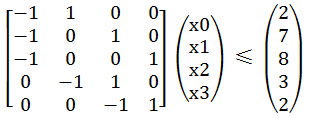然后继续回到单个不等式上来，观察 x[i] - x[j] <= a[k]， 将这个不等式稍稍变形，将x[j]移到不等式右边，则有x[i] <= x[j] + a[k]，然后我们令a[k] = w(j, i)，再将不等式中的i和j变量替换掉，i = v， j = u，将x数组的名字改成d（以上都是等价变换，不会改变原有不等式的性质），则原先的不等式变成了以下形式：d[u] + w(u, v) >= d[v]。

这时候联想到SPFA中的一个松弛操作：

if(d[u] + w(u, v) < d[v]) {
d[v] = d[u] + w(u, v);
}

对比上面的不等式，两个不等式的不等号正好相反，但是再仔细一想，其实它们的逻辑是一致的，因为SPFA的松弛操作是在满足小于的情况下进行松弛，力求达到d[u] + w(u, v) >= d[v]，而我们之前令a[k] = w(j, i)，所以我们可以将每个不等式转化成图上的有向边：

对于每个不等式 x[i] - x[j] <= a[k]，对结点 j 和 i 建立一条 j -> i的有向边，边权为a[k]，求x[n-1] - x 的最大值就是求 0 到n-1的最短路。图三-1-2 展示了 图三-1-1的不等式组转化后的图。

2、三角不等式

如果还没有完全理解，我们可以先来看一个简单的情况，如下三个不等式：

B - A <= c      (1)

C - B <= a      (2)

C - A <= b      (3)

我们想要知道C - A的最大值，通过(1) + (2)，可以得到 C - A <= a + c，所以这个问题其实就是求min{b, a+c}。将上面的三个不等式按照 三-1 数形结合 中提到的方式建图，如图三-2-1所示。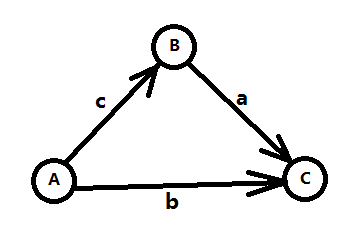我们发现min{b, a+c}正好对应了A到C的最短路，而这三个不等式就是著名的三角不等式。将三个不等式推广到m个，变量推广到n个，就变成了n个点m条边的最短路问题了。

3、解的存在性

上文提到最短路的时候，会出现负权圈或者根本就不可达的情况，所以在不等式组转化的图上也有可能出现上述情况，先来看负权圈的情况，如图三-3-1，下图为5个变量5个不等式转化后的图，需要求得是X[t] - X[s]的最大值，可以转化成求s到t的最短路，但是路径中出现负权圈，则表示最短路无限小，即不存在最短路，那么在不等式上的表现即X[t] - X[s] <= T中的T无限小，得出的结论就是 X[t] - X[s]的最大值 不存在。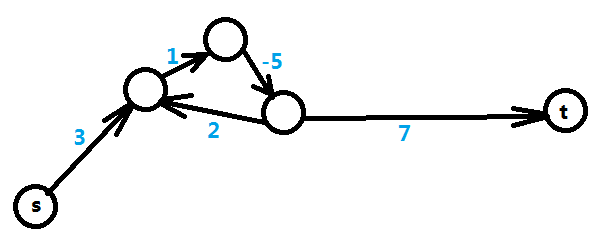再来看另一种情况，即从起点s无法到达t的情况，如图三-3-2，表明X[t]和X[s]之间并没有约束关系，这种情况下X[t] - X[s]的最大值是无限大，这就表明了X[t]和X[s]的取值有无限多种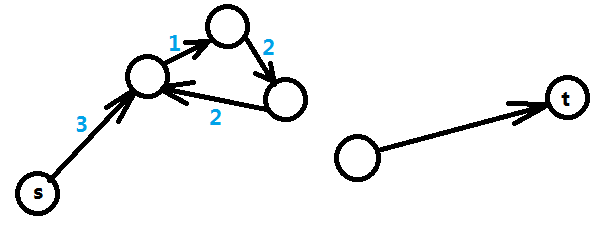在实际问题中这两种情况会让你给出不同的输出。综上所述，差分约束系统的解有三种情况：1、有解；2、无解；3、无限多解；

4、最大值 => 最小值

然后，我们将问题进行一个简单的转化，将原先的"<="变成">="，转化后的不等式如下：

B - A >= c      (1)

C - B >= a      (2)

C - A >= b      (3)

然后求C - A的最小值，类比之前的方法，需要求的其实是max{b, c+a}，于是对应的是图三-2-1从A到C的最长路。同样可以推广到n个变量m个不等式的情况。

5、不等式标准化

如果给出的不等式有"<="也有">="，又该如何解决呢？很明显，首先需要关注最后的问题是什么，如果需要求的是两个变量差的最大值，那么需要将所有不等式转变成"<="的形式，建图后求最短路；相反，如果需要求的是两个变量差的最小值，那么需要将所有不等式转化成">="，建图后求最长路。

如果有形如：A - B = c 这样的等式呢？我们可以将它转化成以下两个不等式：

A - B >= c      (1)

A - B <= c      (2)

再通过上面的方法将其中一种不等号反向，建图即可。

最后，如果这些变量都是整数域上的，那么遇到A - B < c这样的不带等号的不等式，我们需要将它转化成"<="或者">="的形式，即 A - B <= c - 1。

1、线性约束

线性约束一般是在一维空间中给出一些变量（一般定义位置），然后告诉你某两个变量的约束关系，求两个变量a和b的差值的最大值或最小值。

【例题1】N个人编号为1-N，并且按照编号顺序排成一条直线，任何两个人的位置不重合，然后给定一些约束条件。

X(X <= 100000)组约束Ax Bx Cx(1 <= Ax < Bx <= N)，表示Ax和Bx的距离不能大于Cx。

Y(X <= 100000)组约束Ay By Cy(1 <= Ay < By <= N)，表示Ay和By的距离不能小于Cy。

如果这样的排列存在，输出1-N这两个人的最长可能距离，如果不存在，输出-1，如果无限长输出-2。

像这类问题，N个人的位置在一条直线上呈线性排列，某两个人的位置满足某些约束条件，最后要求第一个人和最后一个人的最长可能距离，这种是最直白的差分约束问题，因为可以用距离作为变量列出不等式组，然后再转化成图求最短路。

令第x个人的位置为d[x]（不妨设d[x]为x的递增函数，即随着x的增大，d[x]的位置朝着x正方向延伸）。

那么我们可以列出一些约束条件如下：

1、对于所有的Ax Bx Cx，有 d[Bx] - d[Ax] <= Cx；

2、对于所有的Ay By Cy，有 d[By] - d[Ay] >= Cy；

3、然后根据我们的设定，有 d[x] >= d[x-1] + 1 (1 < x <= N)  （这个条件是表示任何两个人的位置不重合）

而我们需要求的是d[N] - d的最大值，即表示成d[N] - d <= T，要求的就是这个T。

于是我们将所有的不等式都转化成d[x] - d[y] <= z的形式，如下：

1、d[Bx]  -  d[Ax]    <=    Cx

2、d[Ay]  -  d[By]    <=  -Cy

3、d[x-1] -    d[x]    <=    -1

对于d[x] - d[y] <= z，令z = w(y, x)，那么有 d[x] <= d[y] + w(y, x)，所以当d[x] > d[y] + w(y, x)，我们需要更新d[x]的值，这对应了最短路的松弛操作，于是问题转化成了求1到N的最短路。

对于所有满足d[x] - d[y] <= z的不等式，从y向x建立一条权值为z的有向边。

然后从起点1出发，利用SPFA求到各个点的最短路，如果1到N不可达，说明最短路(即上文中的T)无限长，输出-2。如果某个点进入队列大于等于N次，则必定存在一条负环，即没有最短路，输出-1。否则T就等于1到N的最短路。

2、区间约束

【例题2】给定n（n <= 50000）个整点闭区间和这个区间中至少有多少整点需要被选中，每个区间的范围为[ai, bi]，并且至少有ci个点需要被选中，其中0 <= ai <= bi <= 50000，问[0, 50000]至少需要有多少点被选中。

例如3 6 2 表示[3, 6]这个区间至少需要选择2个点，可以是3,4也可以是4,6（总情况有 C(4, 2)种 ）。

这类问题就没有线性约束那么明显，需要将问题进行一下转化，考虑到最后需要求的是一个完整区间内至少有多少点被选中，试着用d[i]表示[0, i]这个区间至少有多少点能被选中，根据定义，可以抽象出 d[-1] = 0，对于每个区间描述，可以表示成d[ bi ]  - d[ ai - 1 ] >= ci，而我们的目标要求的是 d[ 50000 ] - d[ -1 ] >= T 这个不等式中的T，将所有区间描述转化成图后求-1到50000的最长路。

这里忽略了一些要素，因为d[i]描述了一个求和函数，所以对于d[i]和d[i-1]其实是有自身限制的，考虑到每个点有选和不选两种状态，所以d[i]和d[i-1]需要满足以下不等式：  0 <= d[i] - d[i-1] <= 1   （即第i个数选还是不选）

这样一来，还需要加入 50000*2 = 100000 条边，由于边数和点数都是万级别的，所以不能采用单纯的Bellman-Ford ，需要利用SPFA进行优化，由于-1不能映射到小标，所以可以将所有点都向x轴正方向偏移1个单位（即所有数+1）。

3、未知条件约束

未知条件约束是指在不等式的右边不一定是个常数，可能是个未知数，可以通过枚举这个未知数，然后对不等式转化成差分约束进行求解。

【例题3】

i = 0 1 2 3 4 5 6 ... 20 21 22 23 23，分别对应时刻 [i, i+1)，特殊的，23表示的是[23, 0)，并且有些申请者的工作时间可能会“跨天”。

a[i] 表示在第i时刻开始工作的人数，是个未知量

b[i] 表示在第i时刻能够开始工作人数的上限， 是个已知量

R[i] 表示在第i时刻必须值班的人数，也是已知量

那么第i时刻到第i+1时刻还在工作的人满足下面两个不等式（利用每人工作时间8小时这个条件）：

当 i >= 7,        a[i-7] + a[i-6] + ... + a[i] >= R[i]                                     (1)

当 0 <= i < 7,  (a + ... + a[i]) + (a[i+17] + ... + a) >= R[i]              (2)

对于从第i时刻开始工作的人，满足以下不等式：

0 <= i < 24,    0 <= a[i] <= b[i]                                                            (3)

令 s[i] = a + ... + a[i]，特殊地，s[-1] = 0

上面三个式子用s[i]来表示，如下：

s[i] - s[i-8] >= R[i]                               (i >= 7)                                      (1)

s[i] + s - s[i+16] >= R[i]               (0 <= i < 7)                                  (2)

0 <= s[i] - s[i-1] <= b[i]                     (0 <= i < 24)                                (3)

仔细观察不等式(2)，有三个未知数，这里的s就是未知条件，所以还无法转化成差分约束求解，但是和i相关的变量只有两个，对于s的值我们可以进行枚举，令s = T, 则有以下几个不等式：

s[i] - s[i-8] >= R[i]

s[i] - s[i+16] >= R[i] - T

s[i] - s[i-1] >= 0

s[i-1] - s[i] >= -b[i]

对于所有的不等式 s[y] - s[x] >= c，建立一条权值为c的边 x->y，于是问题转化成了求从原点-1到终点23的最长路。

但是这个问题比较特殊，我们还少了一个条件，即：s = T，它并不是一个不等式，我们需要将它也转化成不等式，由于设定s[-1] = 0，所以 s - s[-1] = T，它可以转化成两个不等式：

s - s[-1] >= T

s[-1] - s >= -T

将这两条边补到原图中，求出的最长路s等于T，表示T就是满足条件的一个解，由于T的值时从小到大枚举的（T的范围为0到N），所以第一个满足条件的解就是答案。

最后，观察申请者的数量，当i个申请者能够满足条件的时候，i+1个申请者必定可以满足条件，所以申请者的数量是满足单调性的，可以对T进行二分枚举，将枚举复杂度从O(N)降为O(logN)。

1.Dijkstra

a.邻接矩阵实现（核心代码）

//dis存贮权值  vis是否执行过 mpa用邻接矩阵存贮
void dijkstra(int s)
{
int min_dis,u;  //初始化dis为v行的权值，vis为0
memset(dis,MAX,sizeof(dis));
for(int i=1;i<=n;i++)
{
dis[i]=mpa[s][i];
vis[i]=0;
}
vis[s]=1; //标记第一个v走过
for(int i=1;i<n;i++)//一共需要执行n-1轮
{
min_dis=MAX;
u=-1;
for(int j=1;j<=n;j++)
{
if(!vis[j] && dis[j]<min_dis)
{
u=j;
min_dis=dis[j];
}
}//寻找当前没有走过dis值最小的标号 if(u==-1) break;没有与之相连的点
vis[u]=1;//标记u走过
for(int j=1; j<=n; j++)
if(!vis[j]) dis[j]=min(dis[u]+mpa[u][j],dis[j]);
//比较当前dis[u]+mpa[u][j]是否小于dis[j]，取每次的最小
}
}


b.优先队列优化实现（核心代码）

struct Edge{
int u,v,w;
Edge(int uu,int vv,int ww):u(uu),v(vv),w(ww){ }
};
vector<Edge> edge[maxn];
struct Node{
int Id,W;
bool operator < (const Node &b) const { W>b.W;}
};

{
edge[u].push_back(Edge(u,v,w));
}

void Dijkstra(int s)
{
memset(vis,0,sizeof vis); memset(dis,INF,sizeof dis);
priority_queue<Node> q;
q.push(Node{s,0}),dis[s]=0;
while(!q.empty())
{
Node u=q.top();q.pop();
int id=u.Id;
if(vis[id]) continue;
vis[id]=1;
for(int i=0;i<edge[id].size();i++)
{
if(!vis[edge[id][i].v] && dis[edge[id][i].v]>dis[id]+edge[id][i].w)
{
dis[edge[id][i].v]=dis[id]+edge[id][i].w;
q.push(Node{edge[id][i].v,dis[edge[id][i].v]});
}
}
}
}

2.Bellman-Ford （核心代码）

const int maxn=10010;
const int INF=0x3f3f3f3f;
int n,m,dis;

struct Edge{
int u,v,w;
} edge[maxn<<1];

int main()
{
while(cin>>n>>m,n+m)
{
for(int i=1;i<=2*m;i+=2)
{
cin>>edge[i].u>>edge[i].v>>edge[i].w;
edge[i+1].u=edge[i].v,edge[i+1].v=edge[i].u,edge[i+1].w=edge[i].w;//无向图
}
for(int i=1;i<=n;i++) dis[i]=INF;dis=0;
for(int k=1;k<n;k++)
{
for(int i=1;i<=2*m;i++)
{
if(dis[edge[i].u]<INF)
dis[edge[i].v]=min(dis[edge[i].v],dis[edge[i].u]+edge[i].w);
}
}
/*	//判断是否含有负权回路
bool flag=false;
for(int i=1;i<=2*m;i++)
{
if(dis[edge[i].v]>dis[edge[i].u]+edge[i].w)
{
flag=true; break;
}
}
if(flag) cout<<"Orz,此图含有负权回路"<<endl;
*/
cout<<dis[n]<<endl;
}
return 0;
}

3.SPFA(FIFO队列优化)（核心代码）

const int maxn=10010;
const int INF=0x3f3f3f3f;
struct Edge{
int u,v,w;
Edge(int from,int to,int ww) : u(from),v(to),w(ww) { }
};

vector<Edge> edges;
vector<int> G;
int n,m,u,v,w,vis[maxn<<1],dis,cnt[maxn<<1];
void Init()
{
memset(dis,INF,sizeof dis);dis=0;
memset(vis,0,sizeof vis);
memset(cnt,0,sizeof cnt);
for(int i=0;i<1010;i++) G[i].clear();
edges.clear();
}
{
edges.push_back(Edge(u,v,w));
G[u].push_back(edges.size()-1);
}
bool SPFA(int s)
{
queue<int> q;
q.push(s);
vis[s]=1;
while(!q.empty())
{
int u=q.front();q.pop();
vis[u]=0;
for(int i=0;i<G[u].size();i++)
{
if(dis[u]<INF && dis[edges[G[u][i]].v]>dis[u]+edges[G[u][i]].w)
{
dis[edges[G[u][i]].v]=dis[u]+edges[G[u][i]].w;
if(!vis[edges[G[u][i]].v]){
q.push(edges[G[u][i]].v);vis[edges[G[u][i]].v]=1;
if(++cnt[edges[G[u][i]].v]>n) return false;//Orz有负权回路
}
}
}
}
return true;
}

4.Folyd（核心代码）

    int n,m,u,v,w;
cin>>n>>m;
vector<vector<int> > dis[n];
for(int i=0;i<n;i++) dis[i].resize(n,INF),dis[i][i]=0;
for(int i=0;i<m;i++)
{
cin>>u>>v>>w;
dis[u][v]=w;
//dis[v][u]=w;无向图
}

//核心
for(int k=0;k<n;k++)
{
for(int i=0;i<n;i++)
{
for(int j=0;j<n;j++)
dis[i][j]=min(dis[i][k],dis[k][j]);
}
}posted @ 2018-07-20 01:56  StarHai  阅读(...)  评论(...编辑  收藏